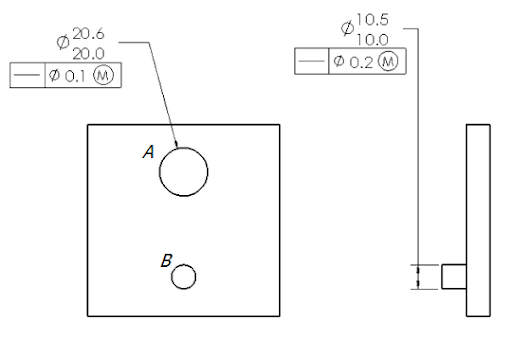Jul 31, 2009

# GD&T Quiz – Virtual Condition, Bonus Tolerance

##### Next ChapterLet us review what we have learned so far. Refer the drawing above and try answering the following questions.

1. What is the virtual condition of feature ‘A’ ?

2. What is the bonus tolerance of feature ‘A’ if actual size is 20.6 ?

3. What is the bonus tolerance of feature ‘A’ if actual size is 20.4 ?

4. What is the bonus tolerance of feature ‘A’ if actual size is 20.0 ?

5. What is the virtual condition of feature ‘B’ ?

6. What is the bonus tolerance of feature ‘B’ if actual size is 10.5 ?

7. What is the bonus tolerance of feature ‘B’ if actual size is 10.1 ?

8. What is the bonus tolerance of feature ‘B’ if actual size is 10.0 ?

1. 19.9

2. 0.6

3. 0.4

4. 0.0

5. 10.7

6. 0.0

7. 0.4

8. 0.5